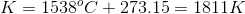# MCAT Physical : Units and Unit Conversion

## Example Questions

### Example Question #1 : Units And Unit Conversion

Which of the following equations is equal to Young's Modulus for a solid?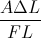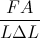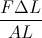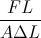Explanation:

Young's Modulus is equal to the stress over strain on an object. The stress on an object refers to the pressure that is put on an object, and can be given the below equation.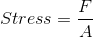The strain on an object refers to the amount an object is deformed in the direction of the force. It can be given the below equation, where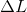is the change in length andis the original length.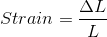Combining these two equations we can determine Young's Modulus.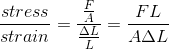### Example Question #2 : Units And Unit Conversion

Which answer choice is the highest temperature?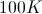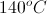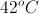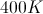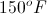Explanation:

To find the right answer, we need to know two formulas: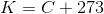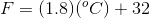The simplest way to compare these choices will be to convert them all to Kelvin.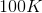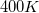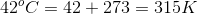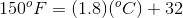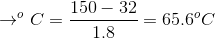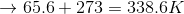This makes our answer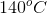.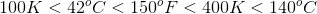### Example Question #3 : Units And Unit Conversion

The freezing point of water is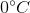and the boiling point of water is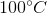. What is the difference between the boiling point and melting point of water if a student measured the temperatures in Fahrenheit?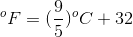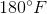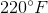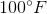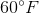Explanation:

In this question, you will have to use the given equation twice; once for melting point and once for boiling point. Let’s first convert the melting point to Fahrenheit. The question states that the melting point of water is. Plugging this into the equation gives: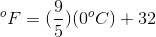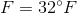The melting point of water is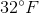. Similarly, we convert the boiling point of water to Fahrenheit: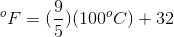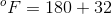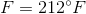The boiling point is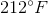. The difference between boiling point and melting point in Fahrenheit is: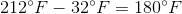### Example Question #4 : Units And Unit Conversion

Which of the following is true regarding the definition of Kelvin?

It is defined so that the temperature at which water exists in all three phases (solid, liquid, and gas) is absolute zero

It is defined as the ratio of the boiling point to the melting point of water

It is defined so that the temperature at which water exists in all three phases (solid, liquid, and gas) is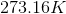It is defined as the ratio of the melting point to the boiling point of water

It is defined so that the temperature at which water exists in all three phases (solid, liquid, and gas) isExplanation:

Kelvin is a temperature scale that is named after Lord Kelvin. Lord Kelvin, through his experiments, found that absolute zerooccurs at a temperature of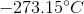. Later, scientists defined Kelvin as the fraction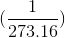of the temperature of water at its triple point (the point on a phase diagram at which water exists as a solid, a liquid, and a gas). This is because the temperature at the triple point of water, in Celsius, is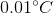. In Kelvin scale, the triple point of water occurs at.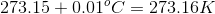### Example Question #5 : Units And Unit Conversion

The melting point of iron is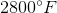. In Celsius the melting point of iron is __________ and in Kelvin it is __________.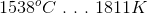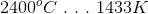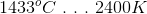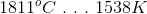Explanation:

The question states that the melting point of iron is. We can use the given equation to convert this to Celsius.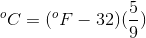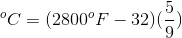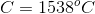This means that the melting point of iron is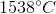. At this point you can choose the right answer because there is only one answer option that has; however, let’s solve for Kelvin. The second equation you need to know is the conversion from Celsius to Kelvin: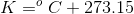The melting point of iron in Kelvin is: